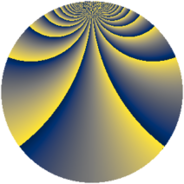# Properties

 Label 507.2.tLevel $507$ Weight $2$ Character orbit 507.t Rep. character $\chi_{507}(4,\cdot)$ Character field $\Q(\zeta_{78})$ Dimension $696$ Newform subspaces $2$ Sturm bound $121$ Trace bound $1$

# Related objects

## Defining parameters

 Level: $$N$$ $$=$$ $$507 = 3 \cdot 13^{2}$$ Weight: $$k$$ $$=$$ $$2$$ Character orbit: $$[\chi]$$ $$=$$ 507.t (of order $$78$$ and degree $$24$$) Character conductor: $$\operatorname{cond}(\chi)$$ $$=$$ $$169$$ Character field: $$\Q(\zeta_{78})$$ Newform subspaces: $$2$$ Sturm bound: $$121$$ Trace bound: $$1$$ Distinguishing $$T_p$$: $$2$$

## Dimensions

The following table gives the dimensions of various subspaces of $$M_{2}(507, [\chi])$$.

Total New Old
Modular forms 1512 696 816
Cusp forms 1416 696 720
Eisenstein series 96 0 96

## Trace form

 $$696 q - q^{3} - 26 q^{4} + 3 q^{7} + 29 q^{9} + O(q^{10})$$ $$696 q - q^{3} - 26 q^{4} + 3 q^{7} + 29 q^{9} + 4 q^{10} + 6 q^{11} - 4 q^{12} + 32 q^{13} + 8 q^{14} - 6 q^{15} + 28 q^{16} + 4 q^{17} - 6 q^{19} - 12 q^{20} + 44 q^{22} - 2 q^{23} + 62 q^{25} + 2 q^{27} - 6 q^{28} - 2 q^{29} + 4 q^{30} + 52 q^{31} - 130 q^{32} + 6 q^{33} + 130 q^{34} + 2 q^{35} - 26 q^{36} - 138 q^{38} - 2 q^{39} + 24 q^{40} + 12 q^{41} - 260 q^{42} + 11 q^{43} - 6 q^{45} - 12 q^{48} + 140 q^{49} - 8 q^{51} - 120 q^{52} + 86 q^{53} - 102 q^{55} + 12 q^{56} + 52 q^{58} - 410 q^{59} - 104 q^{60} - 15 q^{61} - 114 q^{62} - 3 q^{63} - 6 q^{65} + 112 q^{66} - 67 q^{67} + 106 q^{68} + 10 q^{69} - 138 q^{71} - 64 q^{74} + 15 q^{75} - 40 q^{76} - 44 q^{77} - 130 q^{78} + 46 q^{79} + 24 q^{80} + 29 q^{81} - 110 q^{82} - 6 q^{84} - 78 q^{85} - 156 q^{86} + 94 q^{87} - 496 q^{88} - 12 q^{89} - 8 q^{90} + 113 q^{91} + 80 q^{92} - 3 q^{93} - 38 q^{94} - 200 q^{95} - 147 q^{97} + O(q^{100})$$

## Decomposition of $$S_{2}^{\mathrm{new}}(507, [\chi])$$ into newform subspaces

Label Dim $A$ Field CM Traces $q$-expansion
$a_{2}$ $a_{3}$ $a_{5}$ $a_{7}$
507.2.t.a $336$ $4.048$ None $$0$$ $$14$$ $$0$$ $$0$$
507.2.t.b $360$ $4.048$ None $$0$$ $$-15$$ $$0$$ $$3$$

## Decomposition of $$S_{2}^{\mathrm{old}}(507, [\chi])$$ into lower level spaces

$$S_{2}^{\mathrm{old}}(507, [\chi]) \cong$$ $$S_{2}^{\mathrm{new}}(169, [\chi])$$$$^{\oplus 2}$$